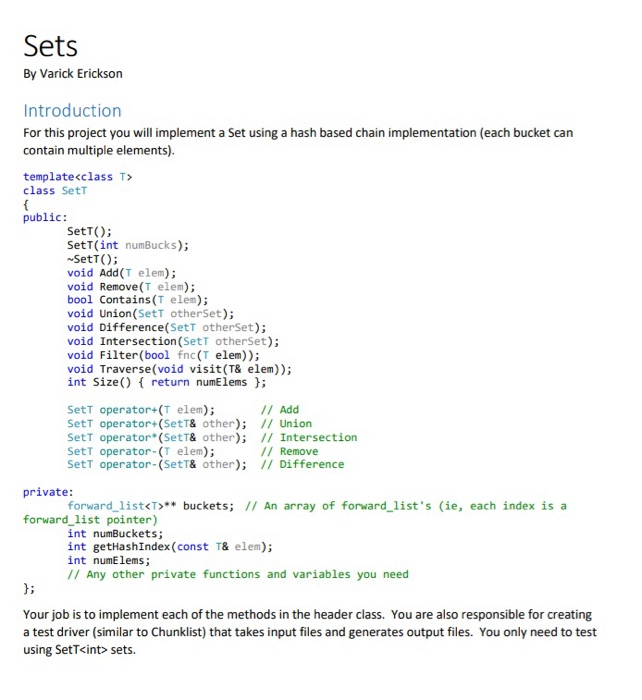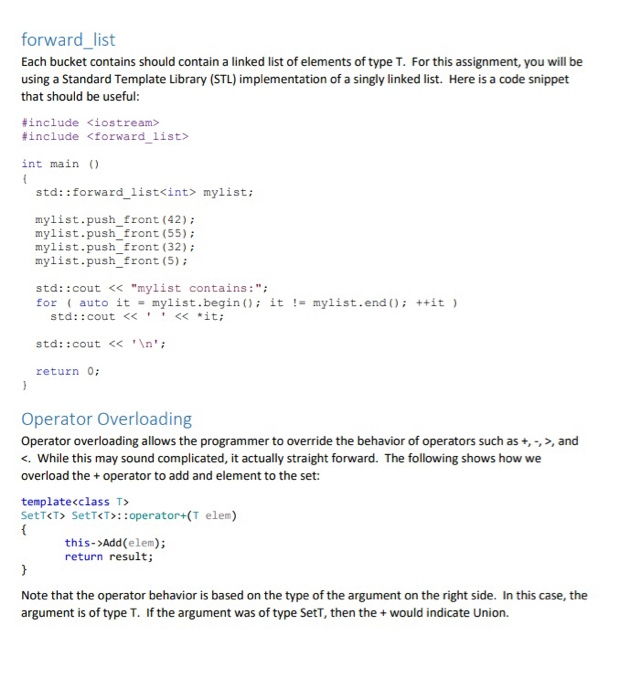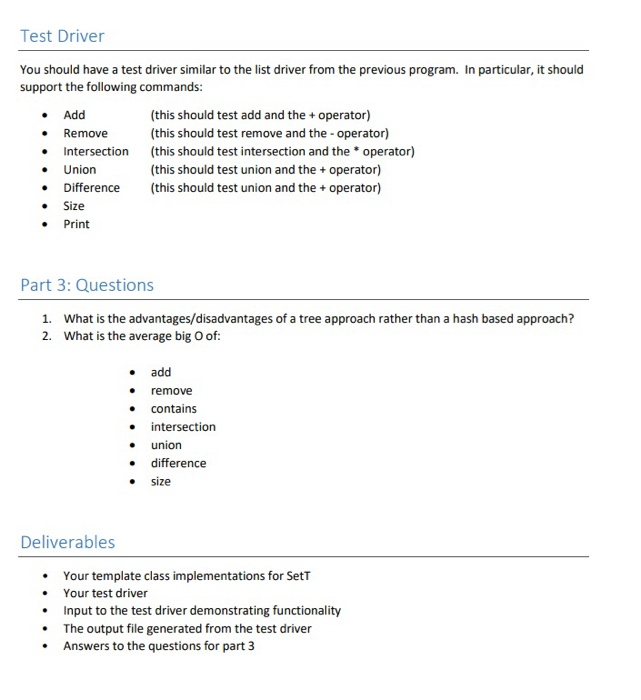# (Solved) : Sets Varick Erickson Introduction Project Implement Set Using Hash Based Chain Implementat Q42577864 . . .

In C++, thanks .Sets By Varick Erickson Introduction For this project you will implement a Set using a hash based chain implementation (each bucket can contain multiple elements). template<class T> class Sett public: Sett(); Sett(int numBucks); Sett(); void Add(T elem); void Remove(T elem); bool Contains (T elem); void Union (Sett otherSet); void Difference(Sett otherSet); void Intersection(Sett otherSet); void Filter(bool fnc(t elem)); void Traverse(void visit(T& elem)); int Size() { return numElems }; Sett operator+(T elem); // Add Sett operator+(SetT& other); // Union Sett operator (Sett& other); // Intersection Sett operator-(T elem); // Remove Sett operator-(Sett& other); // Difference private: forward_list<T>** buckets; // An array of forward_list’s (ie, each index is a forward_list pointer) int numBuckets; int getHashIndex(const T& elem); int numElems; // Any other private functions and variables you need Your job is to implement each of the methods in the header class. You are also responsible for creating a test driver (similar to Chunklist) that takes input files and generates output files. You only need to test using SetT<int sets. forward_list Each bucket contains should contain a linked list of elements of type T. For this assignment, you will be using a Standard Template Library (STL) implementation of a singly linked list. Here is a code snippet that should be useful: #include <iostream> #include <forward list> int main() std:: forward_list int> mylist; mylist.push front (42); mylist.push_front (55); mylist.push front (32); mylist.push front (5); std::cout << “mylist contains: “; for ( auto it = mylist.begin(); it != mylist.end(); ++it) std::cout << ‘ ‘ << *it; std::cout << ‘n’; return 0; Operator Overloading Operator overloading allows the programmer to override the behavior of operators such as +, -, >, and <. While this may sound complicated, it actually straight forward. The following shows how we overload the + operator to add and element to the set: template<class T> Set T<T> SetT<T>:: operator+(T elem) this->Add(elem); return result; Note that the operator behavior is based on the type of the argument on the right side. In this case, the argument is of type T. If the argument was of type Sett, then the + would indicate Union. Test Driver You should have a test driver similar to the list driver from the previous program. In particular, it should support the following commands: Add Remove Intersection Union Difference Size Print (this should test add and the + operator) (this should test remove and the operator) (this should test intersection and the operator) (this should test union and the + operator) (this should test union and the + operator) • • Part 3: Questions 1. What is the advantages/disadvantages of a tree approach rather than a hash based approach? 2. What is the average big o of: add remove contains intersection union difference size Deliverables • • • • • Your template class implementations for Sett Your test driver Input to the test driver demonstrating functionality The output file generated from the test driver Answers to the questions for part 3 Show transcribed image text Sets By Varick Erickson Introduction For this project you will implement a Set using a hash based chain implementation (each bucket can contain multiple elements). template class Sett public: Sett(); Sett(int numBucks); Sett(); void Add(T elem); void Remove(T elem); bool Contains (T elem); void Union (Sett otherSet); void Difference(Sett otherSet); void Intersection(Sett otherSet); void Filter(bool fnc(t elem)); void Traverse(void visit(T& elem)); int Size() { return numElems }; Sett operator+(T elem); // Add Sett operator+(SetT& other); // Union Sett operator (Sett& other); // Intersection Sett operator-(T elem); // Remove Sett operator-(Sett& other); // Difference private: forward_list** buckets; // An array of forward_list’s (ie, each index is a forward_list pointer) int numBuckets; int getHashIndex(const T& elem); int numElems; // Any other private functions and variables you need Your job is to implement each of the methods in the header class. You are also responsible for creating a test driver (similar to Chunklist) that takes input files and generates output files. You only need to test using SetT mylist; mylist.push front (42); mylist.push_front (55); mylist.push front (32); mylist.push front (5); std::cout

Answer to Sets By Varick Erickson Introduction For this project you will implement a Set using a hash based chain implementation (…

We are the best freelance writing portal. Looking for online writing, editing or proofreading jobs? We have plenty of writing assignments to handle.